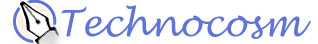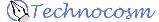Divisibility of Numbers
Have you ever wanted to know some quick tricks to know if a number is divisible by another number? I've always loved shorcuts of this sort, and on a mailing list with some friends, we got to talking about divisibility and I wrote up most of this information at that time.

• Background
When describing the resoning behind these shortcuts, I will ask you to consider numbers of up to four digits, such as 6745. However, I will not use a specific number, instead I will write the number as

1000*a + 100*b + 10*c + d.

A specific number like 6745 is obtained when a=6, b=7, c=4, and d=5. Okay, onto the shortcuts.

• Divisibility by 2
A number is evenly divisible by 2 if its ones digit is evenly divisible by 2.

Why?
Consider a two digit number (10*a+b). Factor this to 2*5*a + b. This shows that all numbers ending a zero are divisible by two, so if the ones digit is divisible by two, the entire number is as well.

• Divisibility by 3
A number is evenly divisible by 3 if the sum of all its digits is evenly divisible by 3.

Why?
Consider a 2 digit number 10*a + b = 9*a + (a+b).

We know that 9*a is divisible by 3, so 10*a + b will be divisible by 3 if and only if a+b is.

Similarly, 100*a + 10*b + c = 99*a + 9*b + (a + b + c), and 99*a + 9*b is divisible by 3, so the total will be divisible by 3 if and only if a + b + c is.

This explanation also works to prove the divisibility by 9 test.

• Divisibility by 4
A number is evenly divisible by 4 if the number formed by the last two individual digits is evenly divisible by 4.

Why?
Consider a three digit number (100*a + 10*b + c)
Factor the first digit: 4*25*a + 10*b + c

Since we know 4*25*a is divisible by 4, we only need to consider 10*b + c, the last two digits, for divisibility by 4.

• Divisibility by 5
A number is evenly divisible by 5 if its ones digit is a 5 or a 0.

Why?
Consider the two digit number (10*a + b)
Factor the first digit: 5*2*a + b

Since 5*2*a is evenly divisible by 5, then only b needs to be evenly divisible by 5, which means b must be 5 or 0.

• Divisibility by 6
A number is evenly divisible by 6 if it is evenly divisible by both 2 and 3.

Why?
Since 2 and 3 are the prime factors of 6, any number with these two prime factors is also divisible by 6.

• Divisibility by 7
Is a number evenly divisible by 7? Take the ones digit off of n, double it and subtract the doubled number from the remaining number. If the result is evenly divisible by 7 (e.g. 14, 7, 0, -7, etc.), then the number is divisible by seven. This process may need to be repeated several times.

Example: 3101. Take off ones digit: 310. Subtract from this the ones digit doubled: 310-2: 308. take off ones: 30. Subtract the ones doubled, which is 16: 14. 14 is divisible by 7, so 3101 is too. It is worth noting that 308 is, of course, also divisible by seven, as are any intermediate results, if and only if N is actually divisible by seven.

Why?
Here is the general case for a three digit number, which is easily extended (I chose three digit numbers, since if we know our times-tables, we already know if all the two-digit numbers are multiples of seven by rote). Consider:

N = (a*100 + b*10 + c).

If we write the inner portion that I have asked you to subtract the multiple from algebraically ("Take the ones digit off of N, double it and subtract the doubled number from the remaining number"), you will see only the terms ( (a*10 +b) - 2*c ) as a partial equation.

At this point, we ask: What must I add to this partial equation to put it back into an expression where N = the original number (a*100 + b*10 + c)? The answer is to multiply the inner portion by 10, put the ones digit back on (add a '+ c') , and then add back 20*c!

N = 10 * ( (a*10 +b) - 2*c) ) + c + 20 * c      =  (a*100 + b*10 + c)

or, after a little reduction:

N = 10 * ( (a*10 +b) - 2*c) ) + 21 * c

We know 21*c is divisible by 7, as we can write it 7*3*c — so this means we only need to know if the inner portion, (a*10 +b) - 2*c), is divisible by seven. This is because 10 * the inner portion will not change the fact that 7 is a prime factor of the inner portion. Either it will be obvious, (i. e., 161 : 16-2 = 14 ) or we can repeat the process until we are satisfied.

• Divisibility by 8
A number is evenly divisible by 8 if the number formed by the last three digits is evenly divisible by 8.

Example: The last three digits of the number 3624 are 624, which is evenly divisible by 8 so 3624 is evenly divisible by 8. Still a little tough for this one.

Why?
Consider the four digit number (1000*a + 100*b + 10*c + d)
Factor the first digit: 8*125*a + 100*b + 10*c + d

Since we know 8*125*a is divisible by 8, we only need to consider 100*b + 10*c + d, the last three digits, for divisibility by 8.

• Divisibility by 9
A number is evenly divisible by 9 if the sum of the individual digits is evenly divisible by 9.

Why?
See the explanation for divisibility by 3.

• Divisibility by 10
A number is evenly divisible by 10 if its ones digit is a 0.

Why?
Consider the two digit number (10*a + b)

Since we know 10*a is evenly divisible by 10, and no non-zero single digit b can be evenly divisible by 10, only if b is zero (causing it to "drop out" of the equation) can 10*a + b be evenly divisible by 10.

• Divisibility by 11
A number is evenly divisible by 11 the alternating sum of its digits are divisible by 11 (including zero).

Example: 82,762,262 is divisible by 11 because:
8-2+7-6+2-2+6-2 = 11, which is divisible by 11.
The rule can also be applied this way: add the total of the odd digits (1st, 3rd, 5th, ...) and subtract the total of the even digits (2nd, 4th, ...)
(8+7+2+6) - (2+6+2+2) = 23 - 12 = 11

Why?
When I get time, I'll write this one up.

• Divisibility by 12
A number is evenly divisible by 12 if it is evenly divisible by both 3 and 4.

Why?
Since 3 and 4 are the prime factors of 12 any number with these two prime factors is also divisible by 12.

• Divisibility by 13
A number is evenly divisible by 13 if, when you delete the last digit from the given number, then subtract nine times the deleted digit from the remaining number, what is left is divisible by 13, then so is the original number. This process may need to be repeated several times.

Example: 1313 is divisible by 13 because:
131-27 = 104, 10-36 = -26. -26 is divisible by 13.

Why?
When I get time, I'll write this one up.

• Divisibility by 14

A number is evenly divisible by 14 if it is evenly divisible by 2 and 7.
Why?
2 and 7 are the prime factors of 14.

• Divisibility by 15

A number is evenly divisible by 15 if it is evenly divisible by 3 and 5.
Why?
3 and 5 are the prime factors of 15.

• Divisibility by n
From here you can easily derive your own rules using prime factors, extensions of powers of two (ie, a number is evenly divisible by 16 if its last four digits are evenly divisible by 16) or a modulus method (ie, for divisibility by 7 and 13) which I have yet to write up. (Of interest: any number whose digits add up to a power of 3 in base 10 are divisible by that power of three...)

• Divisibility by a prime number p
In fact, for any prime p, there exists some integer k such that divisibility by p can be determined by multiplying the units digit by k and adding it to the truncated portion of the numeral.

For example, for the following primes:

p = 7, k = -2
p = 13, k = -9 or 4
p = 17, k = -5
p = 19, k = 2
p = 23, k = 7
p = 29, k = 3
p = 31, k = -3
p = 37, k = -11
p = 41, k = -4
p = 43, k = 13 or -30
p = 47, k = -14

• Divisibility rules in bases other than base 10
There is a cool pair of general proofs using modular arithmetic that say:
1) in base b, a number is divisible by b-1 if and only if its digits added together in base b are divisible by b-1.
2) in base b, a number is divisible by b+1 if and only if the alternating sum of its digits in base b is divisible by b+1.

The proof basically extends the proof of divisibility by 9 and 11 into any base you care to use.

Examples:
511 in base 8 is evenly divisible by 7 in base 8 because 5+1+1 = 7 in base 8.

515 in base 8 is evenly divisible by 11 in base 8 because 5-1+5 = 11 in base 8. (11 in base 8 is written 9 in base 10.)

A12711 in base 12 is evenly divisible by B in base 12 because A+1+2+7+1+1 = 1A in base 12.(B is 11 decimal, 1A is 22 decimal. (not really helpful for you? Sorry!))

Copyright © 2002, 2014 by John Holder